Home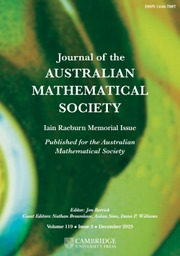Journal of the Australian Mathematical Society

# PRIMITIVE PRIME DIVISORS AND THE$\mathbf{n}$ TH CYCLOTOMIC POLYNOMIAL

Published online by Cambridge University Press:  04 November 2015

Corresponding

## Abstract

Primitive prime divisors play an important role in group theory and number theory. We study a certain number-theoretic quantity, called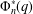$\unicode[STIX]{x1D6F7}_{n}^{\ast }(q)$ , which is closely related to the cyclotomic polynomial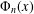$\unicode[STIX]{x1D6F7}_{n}(x)$ and to primitive prime divisors of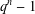$q^{n}-1$ . Our definition of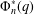$\unicode[STIX]{x1D6F7}_{n}^{\ast }(q)$ is novel, and we prove it is equivalent to the definition given by Hering. Given positive constants$c$ and$k$ , we provide an algorithm for determining all pairs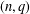$(n,q)$ with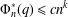$\unicode[STIX]{x1D6F7}_{n}^{\ast }(q)\leq cn^{k}$ . This algorithm is used to extend (and correct) a result of Hering and is useful for classifying certain families of subgroups of finite linear groups.

## MSC classification

Type
Research Article
Information
Journal of the Australian Mathematical Society , February 2017 , pp. 122 - 135
© 2015 Australian Mathematical Publishing Association Inc.

## Access options

Get access to the full version of this content by using one of the access options below.

## References

Bamberg, J. and Penttila, T., ‘Overgroups of cyclic sylow subgroups of linear groups’, Comm. Algebra 36 (2008), 25032543.CrossRefGoogle Scholar
Bang, A. S., ‘Taltheoretiske Undersøgelser’, Tidsskr. Math. (5) 4 (1886), 7080; and 130–137.Google Scholar
Biliotti, M., Jha, V., Johnson, N. L. and Montinaro, A., ‘Translation planes of order q 2 admitting a two-transitive orbit of length q + 1 on the line at infinity’, Des. Codes Cryptogr. 44 (2007), 6986.CrossRefGoogle Scholar
Biliotti, M., Jha, V., Johnson, N. L. and Montinaro, A., ‘Two-transitive groups on a hyperbolic unital’, J. Combin. Theory Ser. A 115 (2008), 526533.CrossRefGoogle Scholar
Biliotti, M., Jha, V., Johnson, N. L. and Montinaro, A., ‘Classification of projective translation planes of order q 2 admitting a two-transitive orbit of length q + 1’, J. Geom. 90(1–2) (2008), 100140.CrossRefGoogle Scholar
Bosma, W., Cannon, J. and Playoust, C., ‘The Magma algebra system. I. The user language’, J. Symbolic Comput. 24(3–4) (1997), 235265.CrossRefGoogle Scholar
Camina, A. R. and Whelan, E. A., Linear Groups and Permutations, Research Notes in Mathematics, 118 (Pitman (Advanced Publishing Program), Boston, MA, 1985).Google Scholar
Dandapat, G. G., Hunsucker, J. L. and Pomerance, C., ‘Some new results on odd perfect numbers’, Pacific J. Math. 57 (1975), 359364.CrossRefGoogle Scholar
DiMuro, J., ‘On prime power order elements of general linear groups’, J. Algebra 367 (2012), 222236.CrossRefGoogle Scholar
Dummit, D. S. and Foote, R. M., Abstract Algebra, 3rd edn, (Wiley & Sons, Hoboken, 2004).Google Scholar
Feit, W., ‘On large Zsigmondy primes’, Proc. Amer. Math. Soc. 102 (1988), 2936.CrossRefGoogle Scholar
The GAP Group, GAP – Groups, Algorithms, and Programming, version 4.7.7; 2015,http://www.gap-system.org.Google Scholar
Glasby, S. P., Supporting GAP and Magma code, http://www.maths.uwa.edu.au/∼glasby/RESEARCH.Google Scholar
Guralnick, R., Penttila, T., Praeger, C. E. and Saxl, J., ‘Linear groups with orders having certain large prime divisors’, Proc. Lond. Math. Soc. 78 (1999), 167214.CrossRefGoogle Scholar
Hering, C., ‘Transitive linear groups and linear groups which contain irreducible subgroups of prime order’, Geom. Dedicata 2 (1974), 425460.CrossRefGoogle Scholar
James, G. D., ‘On the minimal dimensions of irreducible representations of symmetric groups’, Math. Proc. Cambridge Philos. Soc. 94 (1983), 417424.CrossRefGoogle Scholar
Lüneburg, H., ‘Ein einfacher Beweis für den Satz von Zsigmondy über primitive Primteiler von A N - 1. [A simple proof of Zsigmondy’s theorem on primitive prime divisors of A N - 1]’, in: Geometries and Groups (Springer, Berlin, 1981), 219222.CrossRefGoogle Scholar
Niemeyer, A. C. and Praeger, C. E., ‘A recognition algorithm for classical groups over finite fields’, Proc. Lond. Math. Soc. 77 (1998), 117169.CrossRefGoogle Scholar
Ribenboim, P., The Little Book of Big Primes (Springer, New York, 1991).CrossRefGoogle Scholar
Roitman, M., ‘On Zsigmondy primes’, Proc. Amer. Math. Soc. 125 (1997), 19131919.CrossRefGoogle Scholar
Zsigmondy, K., ‘Zur Theorie der Potenzreste’, Monatsh. Math. 3 (1892), 265284.CrossRefGoogle Scholar

### Full text views

Full text views reflects PDF downloads, PDFs sent to Google Drive, Dropbox and Kindle and HTML full text views.

Total number of HTML views: 0
Total number of PDF views: 121 *
View data table for this chart

* Views captured on Cambridge Core between September 2016 - 3rd December 2020. This data will be updated every 24 hours.

Hostname: page-component-b4dcdd7-bf5bq Total loading time: 0.44 Render date: 2020-12-03T18:40:17.151Z Query parameters: { "hasAccess": "0", "openAccess": "0", "isLogged": "0", "lang": "en" } Feature Flags last update: Thu Dec 03 2020 17:59:12 GMT+0000 (Coordinated Universal Time) Feature Flags: { "metrics": true, "metricsAbstractViews": false, "peerReview": true, "crossMark": true, "comments": true, "relatedCommentaries": true, "subject": true, "clr": false, "languageSwitch": true }

# Send article to Kindle

Note you can select to send to either the @free.kindle.com or @kindle.com variations. ‘@free.kindle.com’ emails are free but can only be sent to your device when it is connected to wi-fi. ‘@kindle.com’ emails can be delivered even when you are not connected to wi-fi, but note that service fees apply.

Find out more about the Kindle Personal Document Service.

PRIMITIVE PRIME DIVISORS AND THE$\mathbf{n}$TH CYCLOTOMIC POLYNOMIAL Available formats × # Send article to Dropbox To send this article to your Dropbox account, please select one or more formats and confirm that you agree to abide by our usage policies. If this is the first time you use this feature, you will be asked to authorise Cambridge Core to connect with your <service> account. Find out more about sending content to Dropbox. PRIMITIVE PRIME DIVISORS AND THE$\mathbf{n}$ TH CYCLOTOMIC POLYNOMIAL
Available formats
×

# Send article to Google Drive

To send this article to your Google Drive account, please select one or more formats and confirm that you agree to abide by our usage policies. If this is the first time you use this feature, you will be asked to authorise Cambridge Core to connect with your <service> account. Find out more about sending content to Google Drive.

PRIMITIVE PRIME DIVISORS AND THE$\mathbf{n}\$ TH CYCLOTOMIC POLYNOMIAL
Available formats
×
×